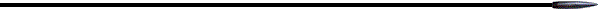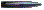Media InfoFAQ## Accurate Versus Low Wind Drift Cartridges

#### By Joe Huffman Copyright 2000Summary of the problemSolutionA  simple problem and it's solutionThe more realistic problemA general formulaDownload the spreadsheetConclusion### Summary of the problem:

Sometimes you have to choose between using an accurate cartridge/firearm and a not so accurate cartridge/firearm but has lower wind drift.  For example one may have a .223 rifle that, under no wind conditions, is capable of 1.0 MOA accuracy.  And you may have a .300 Win Mag that only gets 1.5 MOA of accuracy but is pushing a heavy, high BC, bullet at 2900 fps.  Which one do you choose?  And how do you make the choice if the only criteria is getting the bullet where you want it?

### Solution:

#### A  simple problem and it's solution

Table 1 shows the wind drift in MOA for four different Federal Match cartridges for a 10 MPH, 90 degree, crosswind under Boomershoot conditions (3000 feet above sea level, 70F), for various ranges (in yards).  Using the the Sierra Match King BC's and the Federal specified muzzle velocities -- the .223 has a BC of 0.301 with a MV of 3000 fps, the .308 has a BC of 0.462 with a MV of 2500 fps, the 30.06 has a BC of 0.462 with a MV of 2700 fps, and the .300 Win Mag has a BC of 0.533 with a MV of 2900 fps.

 Cartridge 100 200 300 400 500 600 700 800 900 1000 .223 0.84 1.78 2.81 3.97 5.28 6.76 8.45 10.38 12.48 14.61 .308 0.67 1.39 2.16 3 3.9 4.87 5.93 7.09 8.35 9.72 30.06 0.63 1.31 2.04 2.82 3.67 4.58 5.57 6.65 7.83 9.12 .300 Win 0.49 1.01 1.56 2.15 2.77 3.43 4.14 4.89 5.71 6.58

Table 1. Wind drift in MOA for various cartridges and ranges.

To compute the combined error of windage and rifle inaccuracy you need to add the two errors together in some fashion.  Sometimes the error of the rifle will be in a direction such that it cancels out part or all of the error of the wind.  The result of this can be statistically computed and will be done so later, but initially the extreme spread of the error will be the point of investigation.  To compute the extreme spread we directly add the error of the rifle and the wind drift.  Hence, a 1.0 MOA rifle shooting the .223 cartridge shown in Table 1 at 500 yards with the shooter completely ignoring the 10 MPH crosswind could have a windage error as great as 6.28 MOA or as small as 5.28 MOA.  Or as another example, a 1.5 MOA rifle shooting the .300 Win cartridge at 500 yards under the same conditions would have an error as great as 4.27 MOA or as small as 1.27 MOA.

Obviously, if you completely ignore the presence of the 10 MPH wind the 1.5 MOA rifle shooting any of the 30 caliber cartridges will provide a better one-shot result than the 1.0 MOA rifle shooting the .223 cartridge.  In fact both the 30.06 and .300 Win at 1.5 MOA error will provide better results than a perfectly accurate .223 rifle (1.27 -> 5.17 MOA versus 5.28 MOA error).  But as you reduce the range the perfect .223 rifle becomes the better choice.  This point is at about 350 yards for the 300 Win.  This is determined by where the windage of the .223 cartridge is less than the combined error of the 1.5 MOA .300 Win plus it's windage.  If the .223 rifle has an accuracy of 1.0 MOA then the cross over point where the .223 becomes the better choice is about 150 yards.

Obviously any shooter with even a small amount of experience is not going to completely ignore the 10 MPH crosswind so the results above are not really relevant to real world conditions.  However, they do present a simple problem whose solution can be generalized to solve the more difficult problem.

#### The more realistic problem

The more realistic problem is where the shooter knows the wind is to within some degree of accuracy, say +/- 1 MPH of the actual value, and compensates precisely for the estimated value of the wind.  So it is this wind estimation error that needs to added, in some fashion, to the accuracy error of the rifle.  That is what will now be investigated.

When there are two random source of error being added together the worst case extreme spread of the error will be the simple addition of the extremes as in the solution explored above.  But this assumes the errors always add together in the worst possible manner.  This is not a valid assumption.

The more realistic result is to assume the two error sources are independent and have a normal (bell shaped curve) distribution.  These are valid assumptions for most situations involving wind measurements and rifle accuracy.  The proper way to compute the sum of these two error is to take the square root of the sum of the squares of the errors.

Now we will calculate the errors for the .300 Win and a .223 cartridges in Table 1 while the shooting at 500 yards, in a wind which has been measured to an accuracy of +/- 1 MPH.  The result is a windage ES of one tenth the values shown in Table 1.  This results in a 0.277 MOA ES for the .300 Win and 0.528 MOA for the .223.  If the .300 Win is a 1.5 MOA, ES, rifle then the total ES error of the .300 Win would then be SQRT(0.277 x 0.277 + 1.5 x 1.5) or 1.53 MOA.  If the .223 rifle is accurate to 1.0 MOA, ES, then it's combined error is SQRT(0.528 x 0.528 + 1.0 x 1.0) or 1.13 MOA.  So in this case the .223 is the better choice.  Moving on out to 1000 yards we calculate mean errors for these same rifles under the same conditions as 1.64 MOA for the .300 Win and 1.77 for the .223.  Here, at 1000 yards, the .300 Win is the better choice.  The cross over point occurs at about 900 yards.

#### A general formula

The above example assumes the wind is accurately known and accounted for to within +/- 1 MPH and the rifles are accurate to 1.0 and 1.5 MOA.  What is desired is a general formula that will allow us to specify a wind estimation error in MPH, the accuracy of the two rifles/cartridges and tell us at what wind error estimation value the better wind cartridge is the best choice at a given range.

Many of the following basic equations, but not the target derivation, are from Modern Practical Ballistics by Arthur J. Pejsa.  Please see this excellent book for more details on exterior ballistics.

The wind drift (Wi -- in inches) is a non-trivial function of range (R -- in yards), crosswind velocity (Vw -- in MPH), ballistic coefficient (BC -- available from the bullet manufacture), and muzzle velocity (MV -- in fps).

Let:

F0 = 166 x BCc x SQRT(MV)
BCc = the air temperature and altitude corrected BC.

For normal Boomershoot conditions:

BCc = 1.15 x BC

Given the above, the wind drift Wi is expressed as:

Wi = 79.2 x R x Vw /(MV x (F0/R - 1.5))

The above can be massaged, after using a minor approximation, to yield the windage error in MOA (Ew) as a function of range, MV, Vw and BC:

Ew = 7563 x Vw /(MV x (F0/R - 1.5))

The total error (Etotal) combining both the error of the rifle (Er) and Ew error is:

Etotal = SQRT(Ew2+Er2)

So solving for when the two rifles have the same Etotal will give the crossover point.

SQRT(Ew12 + Er12) = SQRT(Ew22 + Er22)

This simplifies then expands as follows:

Ew12 + Er12 = Ew22 + Er22
Ew12  - Ew22 = Er22 - Er12
(7563 x Vw/(MV1 x (F01/R - 1.5)))2 - (7563 x Vw/(MV2 x (F02/R - 1.5)))2= Er22 - Er12
(7563 x Vw)2 x (1/(MV1 x (F01/R - 1.5))2 - 1/(MV2 x (F02/R - 1.5))2) = Er22 - Er12

Solving for Vw yields:

Vw2 = (Er22 - Er12)/(75632 x (1/(MV1 x (F01/R - 1.5))2 - 1/(MV2 x (F02/R - 1.5))2))
Vw = 1/7563 x SQRT((Er22 - Er12)/(1/(MV1 x (F01/R - 1.5))2 - 1/(MV2 x (F02/R - 1.5))2))

Where for each of the rifles:

BCc = 1.15 x BC
F0 = 166 x BCc x SQRT(MV)
Er = Error of the rifle in MOA.

Using the .223 and .300 cartridges as in the previous examples at a range of 500 yards:

 Cartridge BC MV F0 (calculated) Er .223 .301 3000 3147 1.0 .300 Win .533 2900 5479 1.5

This yields the following for Vw:

Vw = 1/7563 x SQRT((2.25 - 1.0)/(1/(3000 x (3147/500 - 1.5))2 - 1/(2900 x (5479/500 - 1.5))2))
= 1/7563 x SQRT(1.25/(1/206,841,924 - 1/752,306,155))
= 1/7563 x SQRT(356,596,372)
= 18,884/7563
= 2.50 MPH

Hence, if you cannot estimate or measure the wind more accurately than 2.5 MPH then, at 500 yards and beyond, the 1.5 MOA accurate .300 Win is the better choice.

### Conclusion:

An expression for discovering wind estimation error Vw (in MPH) beyond which, at a given range (R), a less accurate but lower wind drift cartridge is the better choice.  The expression is not simple, but the calculation is much easier than testing at the range.  This equation is:

Vw = 1/7563 x SQRT(( Er22 - Er12)/(1/(MV1 x (F01/R - 1.5))2 - 1/(MV2 x (F02/R - 1.5))2))

Where for each of the rifles at Boomershoot conditions:

BCc = 1.15 x BC
F0 = 166 x BCc x SQRT(MV)
Er = Error of the rifle in MOA.
MV = Muzzle velocity in fps.

By putting this equation in a spreadsheet two different cartridges can be quickly compared to determine which is the better choice. Download the spreadsheet here.Email: Joe Huffman
Last updated: April 03, 2009